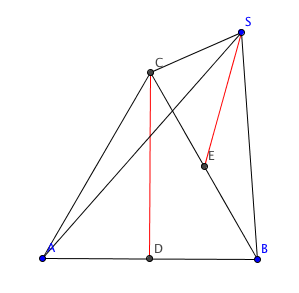# Practice: Find The Distance Between The Skew Lines

Geometry Level 5The base of a pyramid $ABCS$ is an equilateral triangle $ABC$ with a side length of $4\sqrt{2}.$ The edge $SC$ is perpendicular to the base and has length $2.$ Let $D$ and $E$ be the midpoints of $AB$ and $BC$ respectively. The (shortest) distance between the skew lines $SE$ and $CD$ can be written as $\sqrt{\frac{a}{b}},$ where $a$ and $b$ are coprime positive integers. Find $a+b.$

×

Problem Loading...

Note Loading...

Set Loading...